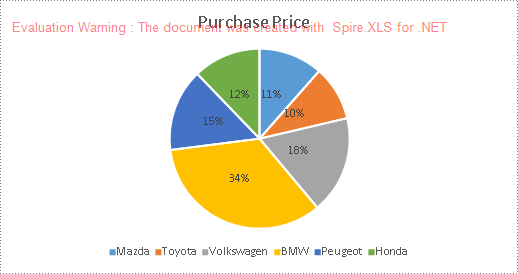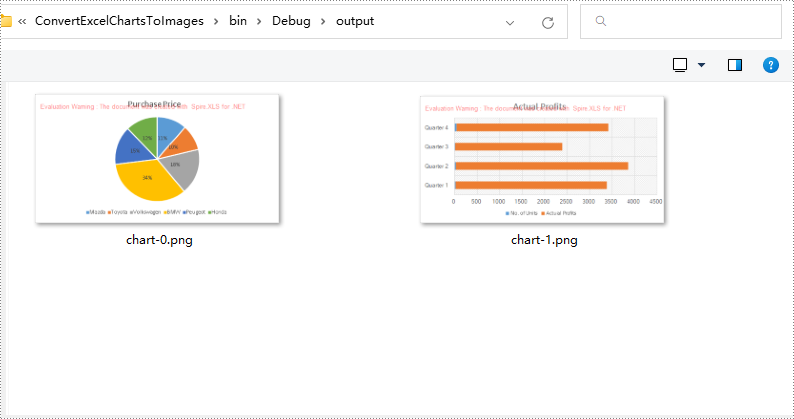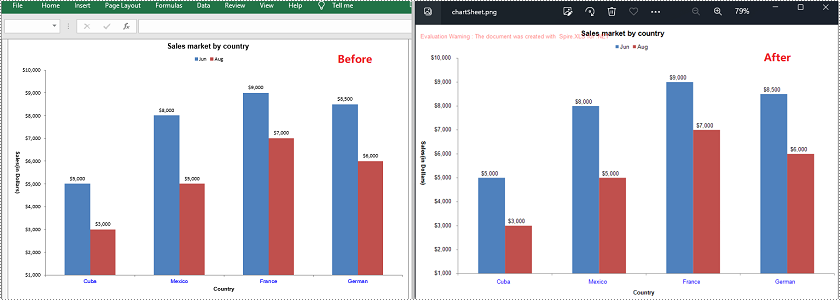# C#/VB.NET: Convert Charts in Excel to Images

Charts are commonly used in Microsoft Excel files to visualize numeric data. In some cases, you may need to save the charts in an Excel file as images in order to use them in other programs or other files such as PDFs and PowerPoint presentations. In this article, we will demonstrate how to convert charts in Excel to images in C# and VB.NET using Spire.XLS for .NET.

### Install Spire.XLS for .NET

To begin with, you need to add the DLL files included in the Spire.XLS for .NET package as references in your .NET project. The DLLs files can be either downloaded from this link or installed via NuGet.

`PM> Install-Package Spire.XLS`

### Convert a Specific Chart in an Excel Worksheet to Image in C# and VB.NET

Spire.XLS provides the Workbook.SaveChartAsImage(Worksheet worksheet, int chartIndex) method which enables you to convert a specific chart in a worksheet as image. The following are the detailed steps:

• Initialize an instance of the Workbook class.
• Get a specific worksheet by its index through Workbook.Worksheets[int worksheetIndex] property.
• Save a specific chart in the worksheet as image using Workbook.SaveChartAsImage(Worksheet worksheet, int chartIndex) method.
• Save the image to a PNG file.
• C#
• VB.NET
```using Spire.Xls;
using System.Drawing;
using System.Drawing.Imaging;

namespace ConvertAExcelChartToImage
{
internal class Program
{
static void Main(string[] args)
{
//Initialize an instance of the Workbook class
Workbook workbook = new Workbook();

//Get the first worksheet
Worksheet sheet = workbook.Worksheets;

//Save the first chart in the first worksheet as image
Image image = workbook.SaveChartAsImage(sheet, 0);
//Save the image to .png file
image.Save(@"output\chart.png", ImageFormat.Png);
}
}
}```### Convert All Charts in an Excel Worksheet to Images in C# and VB.NET

To convert all charts in an Excel worksheet to images, you can use the Workbook.SaveChartAsImage(Worksheet worksheet) method. The following are the detailed steps:

• Initialize an instance of the Workbook class.
• Get a specific worksheet by its index through Workbook.Worksheets[int worksheetIndex] property.
• Save all charts in the worksheet as images using Workbook.SaveChartAsImage(Worksheet worksheet) method.
• Save the images to PNG files.
• C#
• VB.NET
```using Spire.Xls;
using System.Drawing;
using System.Drawing.Imaging;

namespace ConvertAllExcelChartsToImages
{
internal class Program
{
static void Main(string[] args)
{
//Initialize an instance of the Workbook class
Workbook workbook = new Workbook();

//Get the first worksheet
Worksheet sheet = workbook.Worksheets;

//Save charts in the first worksheet as images
Image[] imgs = workbook.SaveChartAsImage(sheet);

//Save the images to png files
for (int i = 0; i < imgs.Length; i++)
{
imgs[i].Save(string.Format(@"output\chart-{0}.png", i), ImageFormat.Png);
}
}
}
}```### Convert a Chart Sheet to Image in Excel in C# and VB.NET

You can use the Workbook.SaveChartAsImage(ChartSheet chartSheet) method to convert a chart sheet in Excel to image. The following are the detailed steps:

• Initialize an instance of the Workbook class.
• Get a specific chart sheet by its index through Workbook.Chartsheets[int chartSheetIndex] property.
• Save the chart sheet as image using Workbook.SaveChartAsImage(ChartSheet chartSheet) method.
• Save the image to .png file.
• C#
• VB.NET
```using Spire.Xls;
using System.Drawing;
using System.Drawing.Imaging;

namespace ConvertExcelChartSheetToImage
{
internal class Program
{
static void Main(string[] args)
{
//Initialize an instance of the Workbook class
Workbook workbook = new Workbook();

//Get the first chart sheet
ChartSheet chartSheet = workbook.Chartsheets;

//Save the first chart sheet as image
Image image = workbook.SaveChartAsImage(chartSheet);
//Save the image to .png file
image.Save(@"output\chartSheet.png", ImageFormat.Png);
}
}
}```### Apply for a Temporary License

If you'd like to remove the evaluation message from the generated documents, or to get rid of the function limitations, please request a 30-day trial license for yourself.